# The Magnetic Force on a Moving Charge The

• Slides: 15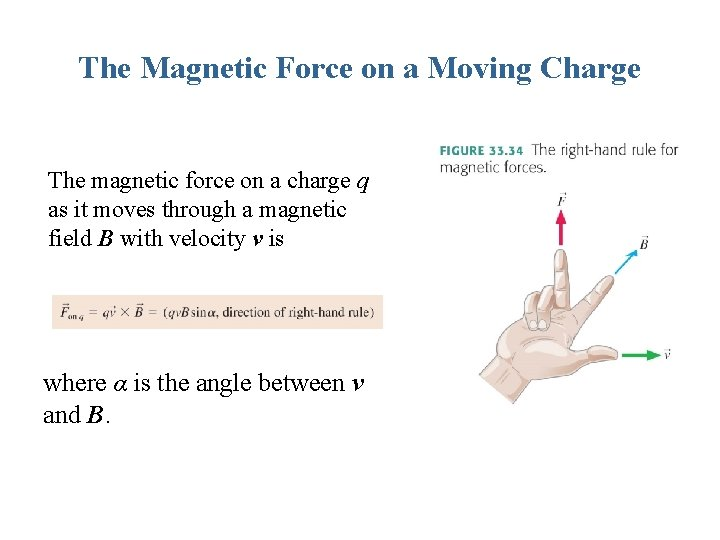The Magnetic Force on a Moving Charge The magnetic force on a charge q as it moves through a magnetic field B with velocity v is where α is the angle between v and B.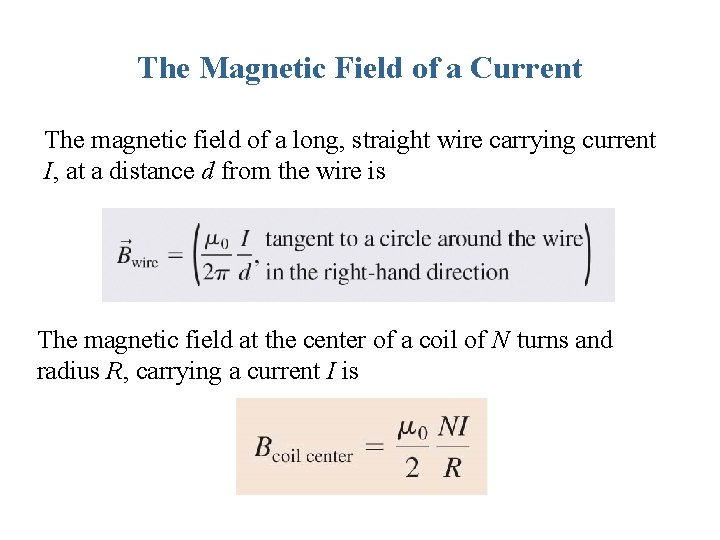The Magnetic Field of a Current The magnetic field of a long, straight wire carrying current I, at a distance d from the wire is The magnetic field at the center of a coil of N turns and radius R, carrying a current I isEXAMPLE 33. 4 The magnetic field strength near a heater wire QUESTION: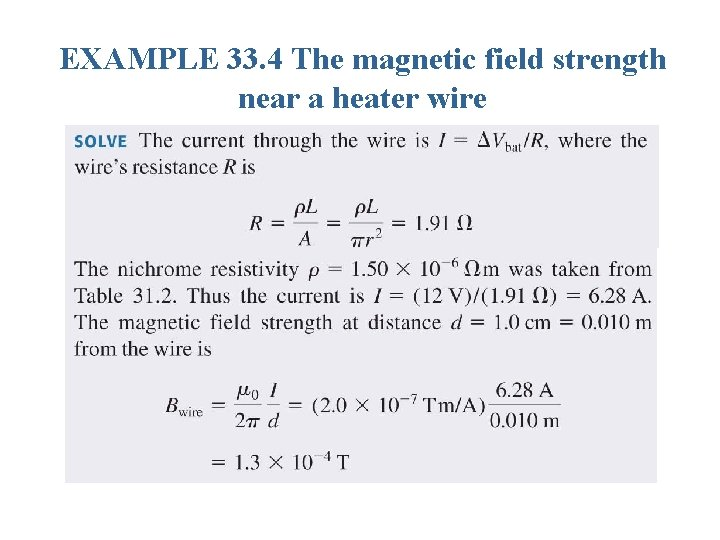EXAMPLE 33. 4 The magnetic field strength near a heater wire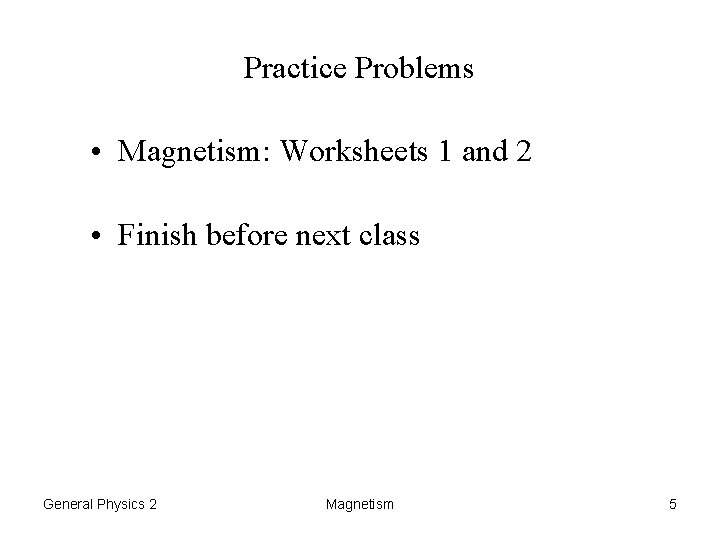Practice Problems • Magnetism: Worksheets 1 and 2 • Finish before next class General Physics 2 Magnetism 5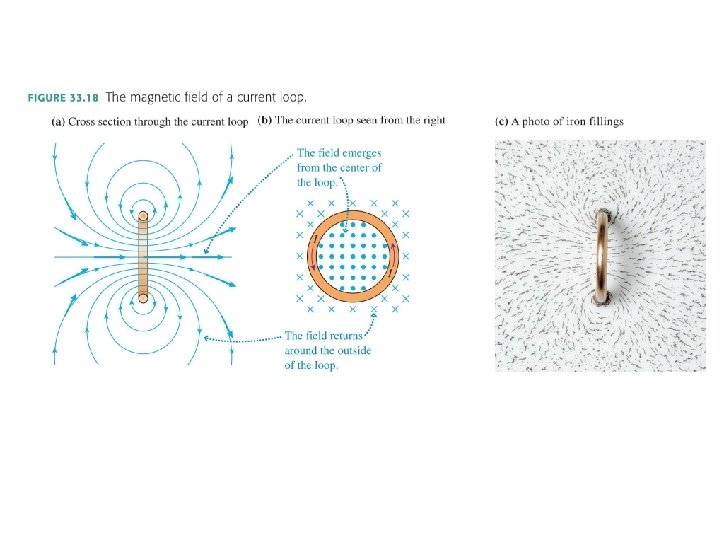Tactics: Finding the magnetic field direction of a current loop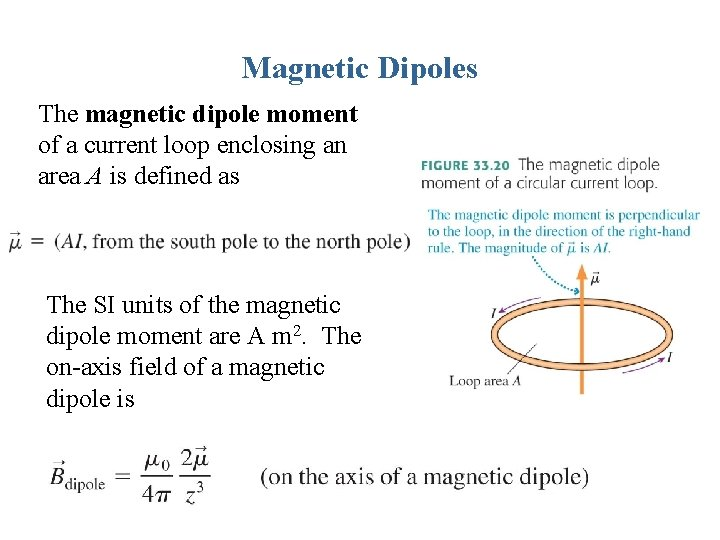Magnetic Dipoles The magnetic dipole moment of a current loop enclosing an area A is defined as The SI units of the magnetic dipole moment are A m 2. The on-axis field of a magnetic dipole isTactics: Evaluating line integralsAmpère’s law Whenever total current Ithrough passes through an area bounded by a closed curve, the line integral of the magnetic field around the curve is given by Ampère’s law:The strength of the uniform magnetic field inside a solenoid is where n = N/l is the number of turns per unit length.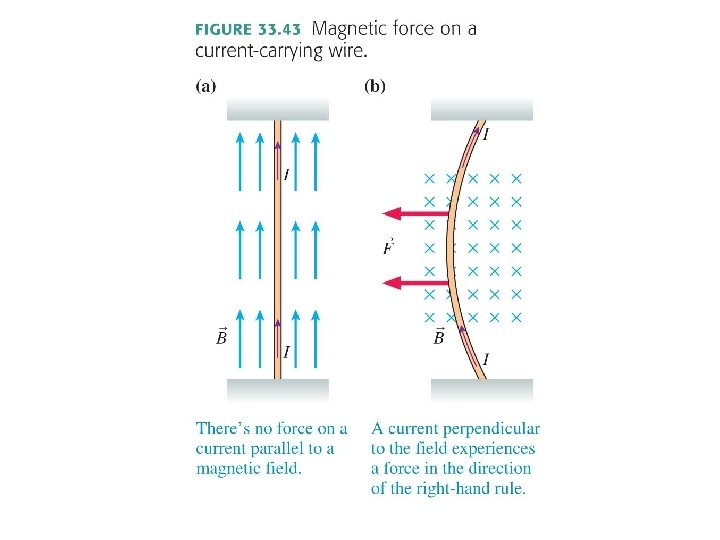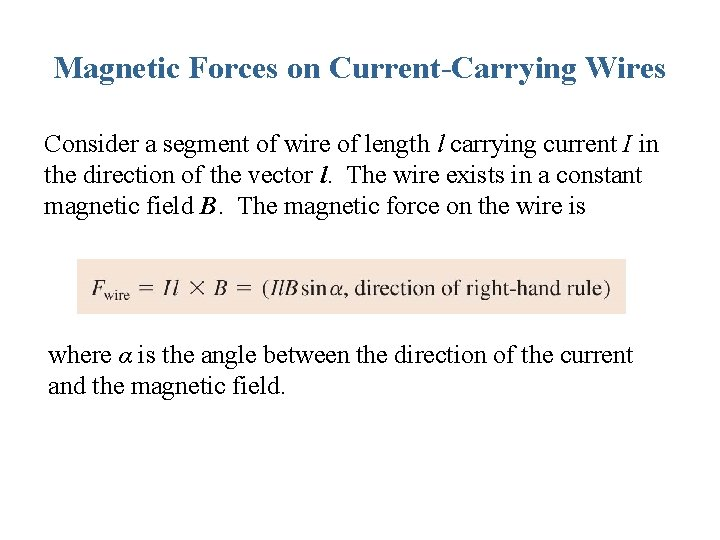Magnetic Forces on Current-Carrying Wires Consider a segment of wire of length l carrying current I in the direction of the vector l. The wire exists in a constant magnetic field B. The magnetic force on the wire is where α is the angle between the direction of the current and the magnetic field.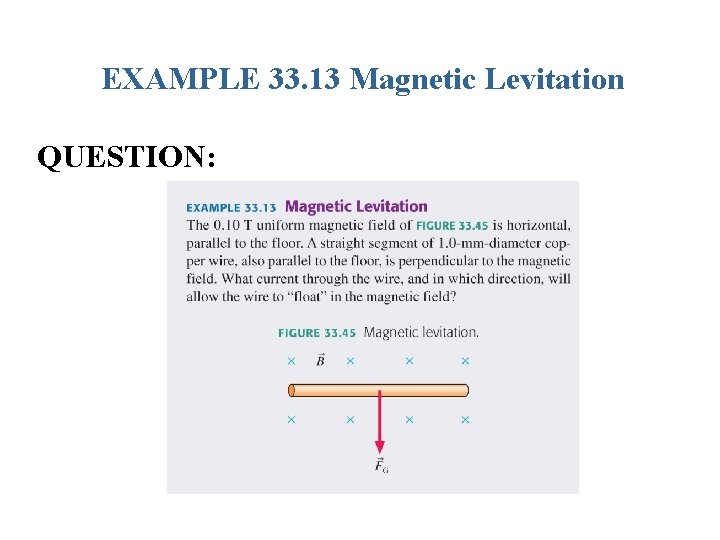EXAMPLE 33. 13 Magnetic Levitation QUESTION:EXAMPLE 33. 13 Magnetic Levitation